# Graph and its representations

Graphs are natural models that are used to represent arbitrary relationships among data objects. A graph is a structure made of two components: a set of vertices V, and a set of edges E. Therefore, a graph is G = (V,E), where G is a graph.  The graph may be directed or undirected. In a directed graph, every edge of the graph is an ordered pair of vertices connected by the edge, whereas in an undirected graph, every edge is an unordered pair of vertices connected by the edge.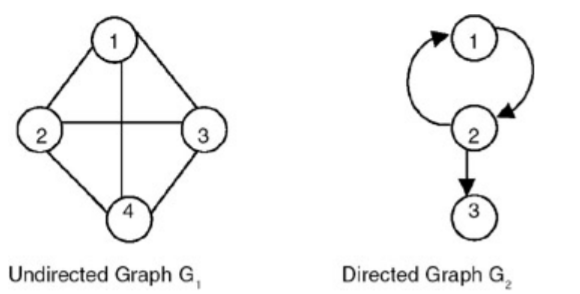Graph is basically a data structure that consists of following two components:
1. A finite set of vertices also called as nodes.
2. A finite set of ordered pair of the form (u, v) called as edge. The pair is ordered because (u, v) is not same as (v, u) in case of directed graph(di-graph). The pair of form (u, v) indicates that there is an edge from vertex u to vertex v. The edges may contain weight/value/cost.

Real life applications of graphs:

Graphs are used to represent networks. The networks may include paths in a city or telephone network or circuit network. Graphs are also used in social networks like linkedIn, facebook. For example, in facebook, each person is represented with a vertex (or node). Each node is a structure and contains information like person id, name, gender and locale.

Important terms in Graphs:

1) Degree of vertex: The number of edges incident onto the vertex. For example, in graph G1, the degree of vertex 1 is 3, because 3 edges are incident onto it. For a directed graph, we need to define indegree and outdegree. Indegree of a vertex vi is the number of edges incident onto vi, with vi as the head. Outdegree of vertex vi is the number of edges incident onto vi, with vi as the tail. For graph G2, the indegree of vertex 2 is 1, whereas the outdegree of vertex 2 is 2.

2)Maximum number of edges: The maximum number of edges in an undirected graph with n vertices is n(n−1)/2. In a directed graph, it is n(n−1).

Following two are the most commonly used representations of graph.
There are other representations also like, Incidence Matrix and Incidence List. The choice of the graph representation is situation specific. It totally depends on the type of operations to be performed and the ease of use.

Adjacency Matrix is a 2D array of size V x V where V is the number of vertices in a graph. Let the 2D array be adj[][], a slot adj[i][j] = 1 indicates that there is an edge from the vertex i to the vertex j. Adjacency matrix for undirected graph is always symmetric. Adjacency Matrix is also used to represent weighted graphs. If adj[i][j] = w, then there is an edge from vertex i to vertex j with weight w.

Example: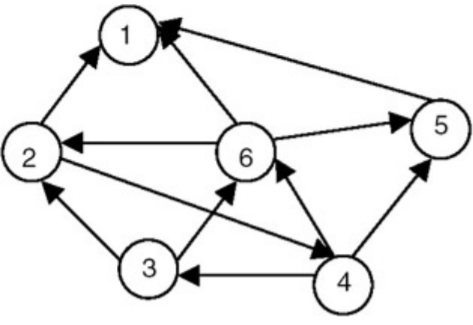The adjacency matrix of the above graph is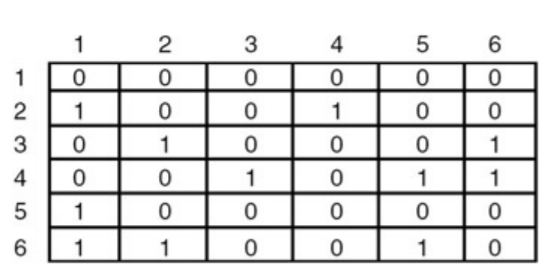Advantages: Representation is easier to implement and follow. Removing an edge takes O(1) time. Queries like whether there is an edge from vertex ‘u’ to vertex ‘v’ are efficient and can be done with O(1) time.

Disadvantages: Consumes more space i.e O(V^2). Even if the graph is sparse (has less number of edges), it consumes the same space. Adding a vertex takes O(V^2) time.

An array of linked lists is used. Size of the array is equal to the number of vertices. Let the array be array[]. An entry array[i] represents the linked list of vertices adjacent to the ith vertex. This representation can also be used to represent a weighted graph. The weights of edges can be stored in nodes of the linked lists. Following is adjacency list representation of the above graph.

Example: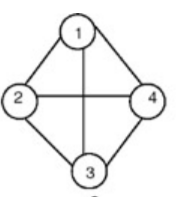The adjacency list representation of the above graph is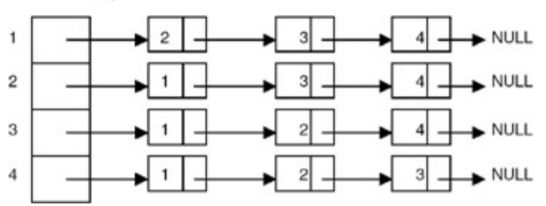Advantages: Saves space O(|V|+|E|) . In the worst case, there can be C(V, 2) number of edges in a graph thus consuming O(V^2) space. Adding a vertex is easier.

Disadvantages: Queries like whether there is an edge from vertex u to vertex v are not efficient and can be done O(V).

Below is the code for adjacency list representation of an undirected graph in C and Java.

## C

```// A C Program to demonstrate adjacency list representation of graphs

#include <stdio.h>
#include <stdlib.h>

// A structure to represent an adjacency list node
{
int dest;
};

// A structure to represent an adjacency list
{
};

// A structure to represent a graph. A graph is an array of adjacency lists.
// Size of array will be V (number of vertices in graph)
struct Graph
{
int V;
};

// A utility function to create a new adjacency list node
{
newNode->dest = dest;
newNode->next = NULL;
return newNode;
}

// A utility function that creates a graph of V vertices
struct Graph* createGraph(int V)
{
struct Graph* graph = (struct Graph*) malloc(sizeof(struct Graph));
graph->V = V;

// Create an array of adjacency lists.  Size of array will be V

// Initialize each adjacency list as empty by making head as NULL
int i;
for (i = 0; i < V; ++i)

return graph;
}

// Adds an edge to an undirected graph
void addEdge(struct Graph* graph, int src, int dest)
{
// list of src.  The node is added at the begining

// Since graph is undirected, add an edge from dest to src also
}

// A utility function to print the adjacenncy list representation of graph
void printGraph(struct Graph* graph)
{
int v;
for (v = 0; v < graph->V; ++v)
{
while (pCrawl)
{
printf("-> %d", pCrawl->dest);
pCrawl = pCrawl->next;
}
printf("\n");
}
}

// Driver program to test above functions
int main()
{
// create the graph given in above fugure
int V = 5;
struct Graph* graph = createGraph(V);

// print the adjacency list representation of the above graph
printGraph(graph);

return 0;
}
```

## Java

```// Java Program to demonstrate adjacency list
// representation of graphs

public class GFG
{
// A user define class to represent a graph.
// A graph is an array of adjacency lists.
// Size of array will be V (number of vertices
// in graph)
static class Graph
{
int V;

// constructor
Graph(int V)
{
this.V = V;

// define the size of array as
// number of vertices

// Create a new list for each vertex
// such that adjacent nodes can be stored
for(int i = 0; i < V ; i++){
}
}
}

// Adds an edge to an undirected graph
static void addEdge(Graph graph, int src, int dest)
{
// Add an edge from src to dest.

// Since graph is undirected, add an edge from dest
// to src also
}

// A utility function to print the adjacency list
// representation of graph
static void printGraph(Graph graph)
{
for(int v = 0; v < graph.V; v++)
{
System.out.println("Adjacency list of vertex "+ v);
System.out.print(" -> "+pCrawl);
}
System.out.println("\n");
}
}

// Driver program to test above functions
public static void main(String args[])
{
// create the graph given in above figure
int V = 5;
Graph graph = new Graph(V);

// print the adjacency list representation of
// the above graph
printGraph(graph);
}
}
```

Output:

``` Adjacency list of vertex 0

head -> 4-> 3-> 2-> 0

### Relevant exercises

POST A NEW COMMENT

• Input (stdin)

Output (stdout)

Input (stdin)

Expected Output

Compiler Message

Input (stdin)

`2    3`

`5`
`5`
`5`